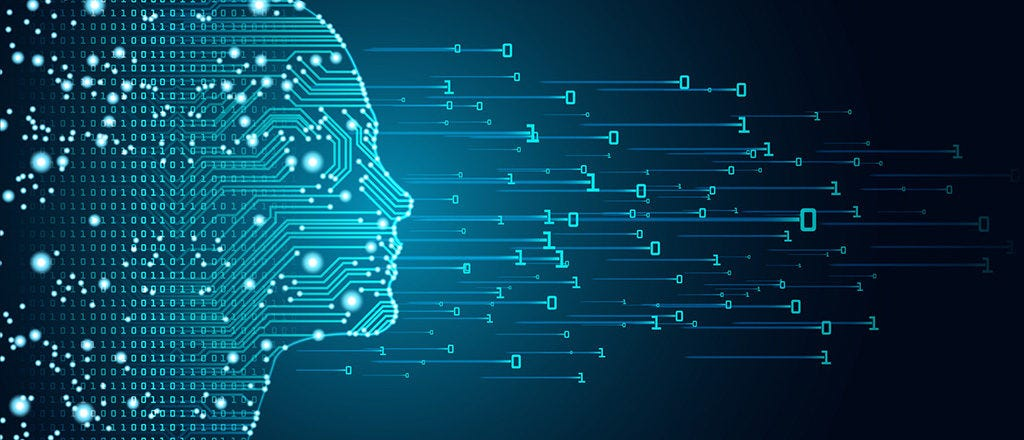# Dimensionality Reduction Techniques in Machine LearningDimensionality Reduction Techniques in Machine Learning. What are Dimensionality Reduction Techniques? Basically, dimension reduction refers to the process of converting a set of data.

## What is Dimensionality Reduction?

In machine learning problems, there are often too many factors on the basis of which the final classification is done. These factors are basically variables called features. The higher the number of features, the harder it gets to visualize the training set and then work on it. The process of selecting a subset of features for use in model construction is called Dimensionality Reduction.

Before Learning the techniques of Dimensionality Reduction, lets understand why it is important to do Dimensionality Reduction in our Dataset.

Reasons :

*1) *The abundance of redundant and irrelevant features

2) With a fixed number of training samples, the predictive power reduces as the dimensionality increases. [_Hughes phenomenon_]

3) Other things being equal, simpler explanations are generally better than complex ones.

*4) *It improves the accuracy of a model if the right subset is chosen.

5) Reduces the Overfitting.

*6) *It reduces computation time.

7) It helps in data compression, and hence reduced storage space.

## Dimensionality Reduction Techniques

1. Percent missing values
2. Amount of variation
3. Multicollinearity
4. Principal Component Analysis (PCA)
5. Correlation (with the target)
6. Forward selection
7. Backward elimination
8. LASSO

## 15 Machine Learning and Data Science Project Ideas with Datasets

Learning is a new fun in the field of Machine Learning and Data Science. In this article, we’ll be discussing 15 machine learning and data science projects.

## Exploratory Data Analysis is a significant part of Data Science

Data science is omnipresent to advanced statistical and machine learning methods. For whatever length of time that there is data to analyse, the need to investigate is obvious.

## Most popular Data Science and Machine Learning courses — July 2020

Most popular Data Science and Machine Learning courses — August 2020. This list was last updated in August 2020 — and will be updated regularly so as to keep it relevant

## Statistics for Data Science

Statistics for Data Science and Machine Learning Engineer. I’ll try to teach you just enough to be dangerous, and pique your interest just enough that you’ll go off and learn more.

## Difference between Machine Learning, Data Science, AI, Deep Learning, and Statistics

In this article, I clarify the various roles of the data scientist, and how data science compares and overlaps with related fields such as machine learning, deep learning, AI, statistics, IoT, operations research, and applied mathematics.